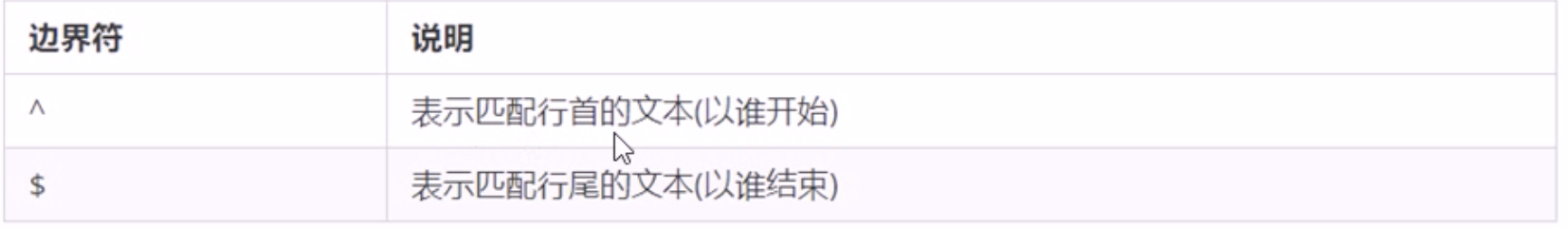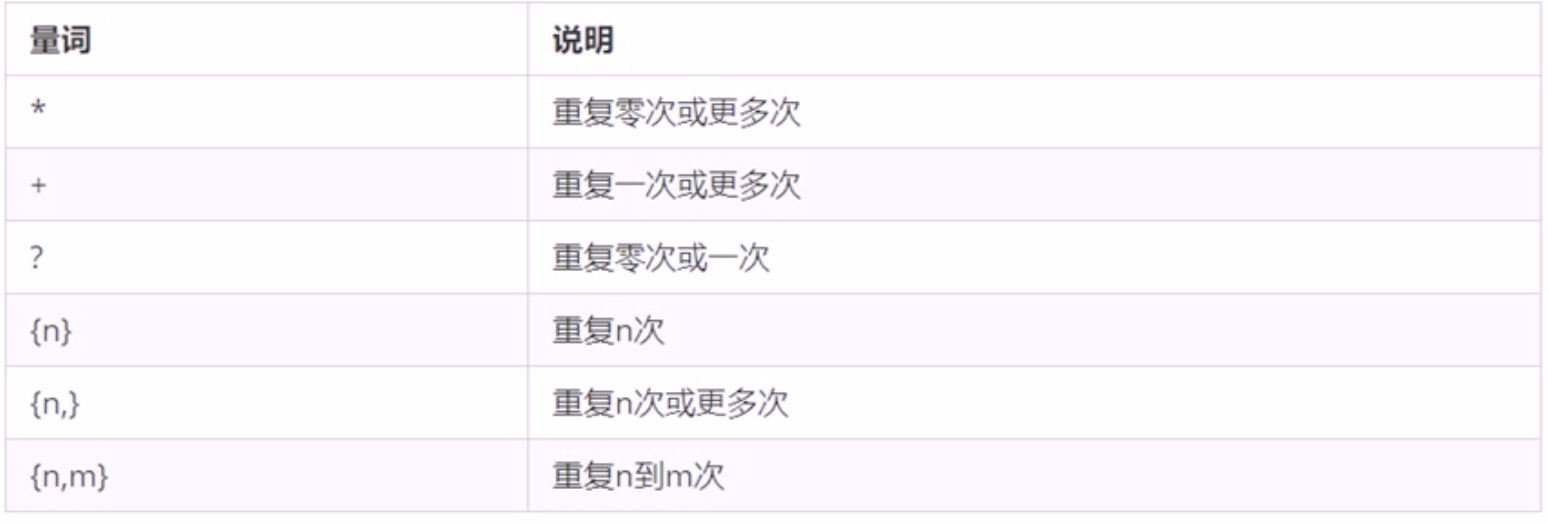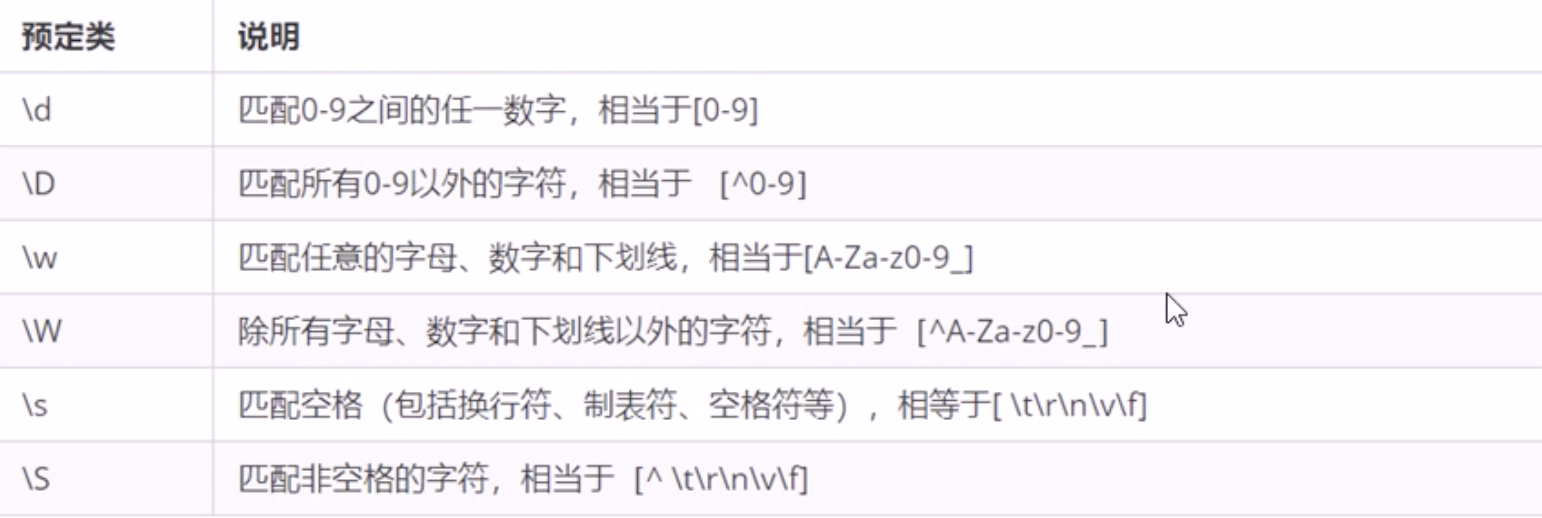## Teach you to easily handle the regular in JavaScript

Tell me Zhan to hide 2020-11-06 20:37:32
teach easily handle regular javascript

### 1 Overview of regular expressions

#### 1.1 What is regular expression

Regular expressions （Regular Expression） Is a pattern used to match character combinations in strings . stay Javascript in , Regular expressions are also objects .
Regular tables are usually used to retrieve , Replace those that match a pattern （ The rules ） The text of , For example, validation forms ： Only English letters can be entered in the user name form 、 Numbers or underscores , Enter your nickname in Chinese （ matching ）. Besides , Regular expressions are also often used to filter out sensitive words in the content of a page , Or get the specific part we need from the string （ extract ） etc. .

#### 1.2 Regular expression features

1. flexibility 、 Very logical and functional
2. You can quickly achieve complex control of strings in extremely simple ways
3. It's hard to understand

### 2 The regular expression is in Javascript The use of

#### 2.1 Create regular expression

1. adopt RegExp Object's constructor creation
``var Variable name = new RegExp(/ expression /)``
``````// utilize RegExp Create objects
var regexp = new RegExp(/123/)
console.log(regexp)``````
1. Create... By literals
``var Variable name = / expression /``
``````var rg = /123/
console.log(rg)``````

#### 2.2 Test regular expressions test

test() Regular object methods , Used to detect whether a string conforms to this rule , The object will return true or false, Its parameter is the test string .

``regexObj.test(str)``
• regexObj It's a regular expression written
• str The text we're going to test
• It's testing str Whether the text conforms to our regular expression specification
``````var rg = /123/
console.log(rg.test(123)) //true
console.log(rg.test('abc')) //false ``````

### 3 Special characters in regular expressions

#### 3.1 The composition of regular expressions

A regular expression can be made up of simple characters , such as /abc/, It can also be a combination of simple and special characters , such as /ab*c/. Special characters are also called metacharacters , In regular expressions, they are special symbols with special meanings .
Because there are many special characters , I'm not going to list them here , You can refer to ：
MDN：https://developer.mozilla.org/zh-CN/docs/Web/JavaScript/Guide/Regular_Expressions
Here we can divide metacharacters into several types of learning ：

#### 3.2 Border character

Boundary characters in regular expressions （ Position symbol ） Used to indicate the position of a character , There are mainly two characters .If ^ and \$ At the same time , Then the exact match is achieved

``````var rg= /abc/ // There's no need for quotation marks in regular expressions
// /abc/ As long as it contains abc This string returns true
console.log(rg.test('abc')) // true
console.log(rg.test('abcd')) // true
console.log(rg.test('aabcd')) // true
var reg = /^abc/
console.log(rg.test('abc')) // true
console.log(rg.test('abcd')) // true
console.log(rg.test('aabcd')) // false
var reg = /^abc\$/ // Exactly match , The requirement must be abc Only strings conform to the specification
console.log(rg.test('abc')) // true
console.log(rg.test('abcd')) // false
console.log(rg.test('aabcd')) // false
console.log(rg.test('abcabc')) // false``````

#### 3.3 Character class

Indicates that there is a series of characters to choose from , Just match one of them , All available characters are enclosed in square brackets

``````var rg = /[abc]/; // As long as it contains a perhaps b perhaps c All back to true
console.log(rg.test('andy')) //true
var rg = /[abc]/; // As long as it contains a perhaps b perhaps c All back to true
console.log(rg.test('baby')) //true
console.log(rg.test('color')) //true
console.log(rg.test('red')) //false
var rg = /^[abc]\$/ // Choose one of three Only a Or is it b Or is it c, These three letters return to true
console.log(rg.test('aa')) //false
console.log(rg.test('a')) //true
console.log(rg.test('b')) //true
console.log(rg.test('c')) //true
console.log(rg.test('abc')) //false
var rg = /^[a-z]\$/ //26 English letters, any letter returns true
var rg = /^[a-zA-Z]\$/ //26 English letters ( Upper case and lower case ) Any letter returns true
var rg = /^[a-zA-Z0-9_-]\$/
console.log(rg.test('c')) //true
console.log(rg.test('B')) //true
console.log(rg.test('_')) //true
console.log(rg.test('8')) //true
console.log(rg.test('-')) //true
// If there is ^, It means to take the opposite , To match the border ^ Don't confuse
var rg = /^[^a-zA-Z]\$/``````

#### 3.4 Quantifier sign

Quantifier is used to set the number of times a pattern appears``````var reg = /^a*\$/
console.log(reg.test(''))// true
console.log(reg.test('a'))// true
console.log(reg.test('aaa'))// true
var reg = /^a+\$/
console.log(reg.test(''))// false
console.log(reg.test('a'))// true
console.log(reg.test('aaa'))// true
var reg = /^a?\$/
console.log(reg.test(''))// true
console.log(reg.test('a'))// true
console.log(reg.test('aaa'))// false
var reg = /^a{3}\$/
console.log(reg.test(''))// false
console.log(reg.test('a'))// false
console.log(reg.test('aaa'))// true
var reg = /^a{3,}\$/
console.log(reg.test(''))// false
console.log(reg.test('a'))// false
console.log(reg.test('aaa'))// true
console.log(reg.test('aaaaa'))// true
var reg = /^a{
3,5}\$/ //{3,5} Don't leave a space after a comma
console.log(reg.test(''))// false
console.log(reg.test('a'))// false
console.log(reg.test('aaa'))// true
console.log(reg.test('aaaaa'))// true``````

User name verification

1. If the user name is legal , Then the following prompt message is ： The user name is legal , And the color is green
2. If the user name is not legal , Then the following prompt message is ： The user name does not conform to the specification , And the color is green

User name authentication analysis ：

1. The user name can only be in English letters , Numbers , An underline or dash , And the length of the user name is 6~16 position .
2. First prepare this regular expression pattern
3. If it's a regular specification , Let's have the following span add right class .
4. If it doesn't conform to the regular specification , Let's have the following span add wrong class .
``````<input type="text" class="uname"> <span> Please enter a user name </span>
<style> span {  color: #aaa; font-size: 14px; } .right {  color: green; } .wrong {  color: red; } </style>``````
``````var reg=/^[a-zA-Z0-9-_]{6,16}\$/
var uname = doucument.querySelector('.uname');
var spn = doucument.querySelector('span');
uname.onblur = function() {

if(res.test(this.value)){

span.className='right'
span.innerHTML = ' The user name input format is correct '
} else {

span.className='wrong'
span.innerHTML = ' User name input format error '
}
}``````

#### 3.5 Brackets summarize

1. Curly braces ： Quantifier sign It means the number of repetitions
2. brackets ： Character set . Match any character in the formula bracket
3. parentheses ： Express priority
``````// brackets
var reg = /^[abc]\$/ //a||b||c
// Curly braces
var reg = /^abc{
3}\$/ // It just makes c Repeat three times
// parentheses
var reg = /^(abc){
3}\$/ //abc Repeat three times ``````

#### 3.6 Predefined classes

Predefined class refers to the abbreviation of some common patternsLandline number verification

``````var reg= /^\d{
3}-\d{
8}|\d{
4}-\d{
7}\$/ // | It means or means
var reg= /^\d{3,4}-\d{7,8}|\d{4}-\d{7}\$/``````

### 4 Substitution in regular expressions

#### 4.1 replace Replace

replace() Method can implement the replacement string operation , The replacement parameter can be a string or a regular expression .

``stringObject.replace(regxp/substr, replacement)``
1. The first parameter ： Replaced string or regular expression
2. The second parameter ： Replace with a string of
3. The return value is a new string after replacement
``````var str = 'lanfeng and qianduan'
var newStr = str.replace('front', 'qianduan')
console.log(newstr)``````

Message filtering sensitive words

``<textarea name="" id="message"></textarea> <button> Submit </button>``
``````var text = document.querySelector('textarea');
var btn = document.querySelector('button');
var div = doucment.querySelector('div');
btn.onclick = function () {

div.innerHTML = text.value.replace(/ passion /g, '**')
}``````

#### 4.2 Regular expression parameters

``/ expression /[switch]``

switch（ Also known as a modifier ） According to what pattern to match , There are three values

• g: The global matching
• i： Ignore case
• gi: The global matching + Ignore case

### summary

This article mainly shares javascript Some knowledge points and usage of middle regular .